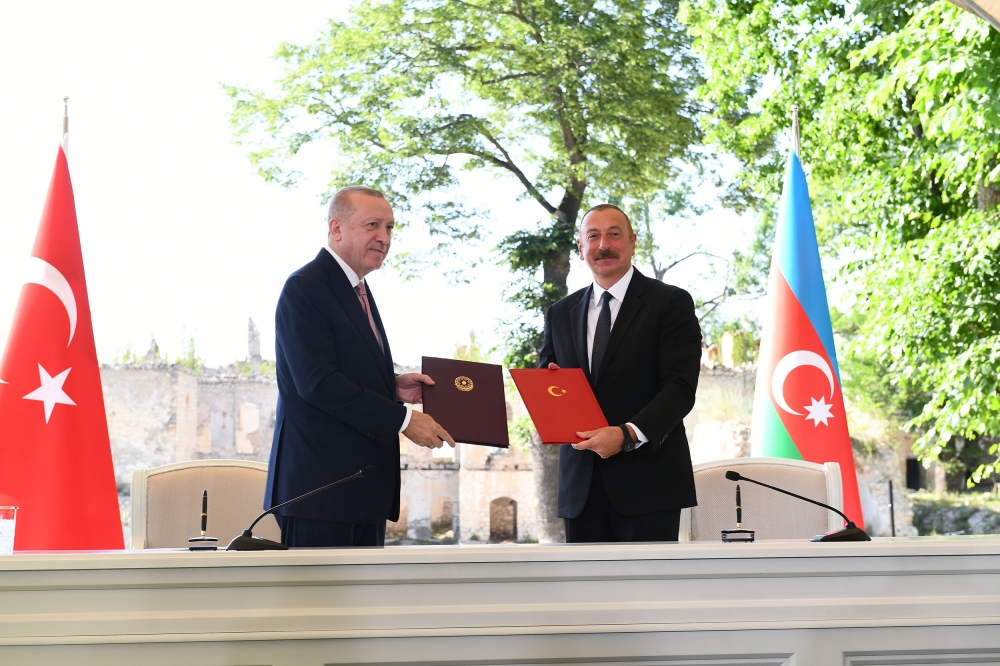Institute of Mathematics and Mechanics
Azerbaijan National Academy of Science

## Karabakh news### “Musical heritage and Karabakh horses on Jidir Duzu plain” composition organized by Heydar Aliyev Foundation was presented in Shusha### Azerbaijan, Turkey signed Shusha Declaration on allied relations## Ismayilov Vugar Elman

Basic scientific achievements

1) Necessary and sufficient conditions for the representation of multivariate functions by linear combinations of ridge functions were obtained;

2) A Chebyshev type theorem was proved for sums of ridge functions to be extremal to a given continuous function;

3) Explicit formulas for an exact computation of the approximation error and construction of best approximating function were obtained in problems of approximation of multivariate functions by ridge functions and univariate functions in both continuous and square-integrable metrics;

4) It was shown that if each continuous function defined on a compact Hausdorff space is represented by linear superpositions, then all functions on this space possess such representation;

5) The problem of multivariate approximation theory associated with Golomb’s theorem was solved.

The list of publications at the 5 years published

1. (with N. Guliyev) On the approximation by single hidden layer feedforward neural networks with fixed weights, Neural Networks98(2018), 296-304, https://doi.org/10.1016/j.neunet.2017.12.007
2. A note on the criterion for a best approximation by superpositions of functions, Studia Mathematica240 (2018), no. 2, 193-199, https://doi.org/10.4064/sm170314-9-4
3. (with A. Asgarova) On the representation by sums of algebras of continuous functions, Comptes Rendus Mathematique355 (2017), no. 9, 949-955, https://doi.org/10.1016/j.crma.2017.09.015
4. A note on the equioscillation theorem for best ridge function approximation, Expositiones Mathematicae35 (2017), no. 3, 343-349, https://doi.org/10.1016/j.exmath.2017.05.003
5. (with A. Asgarova) Diliberto–Straus algorithm for the uniform approximation by a sum of two algebras, Proceedings – Mathematical Sciences127 (2017), no. 2, 361-374, http://dx.doi.org/10.1007/s12044-017-0337-4
6. (with E. Savas) Measure theoretic results for approximation by neural networks with limited weights, Numerical Functional Analysis and Optimization38(2017), no. 7, 819-830, http://dx.doi.org/10.1080/01630563.2016.1254654
7. Approximation by sums of ridge functions with fixed directions, (Russian) Algebra i Analiz 28 (2016), no. 6, 20–69, http://mi.mathnet.ru/eng/aa1513 English transl. St. Petersburg Mathematical Journal 28 (2017), 741-772, https://doi.org/10.1090/spmj/1471
1. On the uniqueness of representation by linear superpositions, Ukrainskii Matematicheskii Zhurnal68(2016), no. 12, 1620-1628. English transl. Ukrainian Mathematical Journal 68 (2017), no. 12, 1874-1883, https://doi.org/10.1007/s11253-017-1335-5
2. (with N. Guliyev) A single hidden layer feedforward network with only one neuron in the hidden layer can approximate any univariate function, Neural Computation 28(2016), no. 7, 1289–1304, http://dx.doi.org/10.1162/NECO_a_00849
3. (with R. Aliev) On a smoothness problem in ridge function representation, Advances in Applied Mathematics73(2016), 154–169, http://dx.doi.org/10.1016/j.aam.2015.11.002
4. Approximation by ridge functions and neural networks with a bounded number of neurons, Applicable Analysis94(2015), no. 11, 2245-2260, http://dx.doi.org/10.1080/00036811.2014.979809
5. On the approximation by neural networks with bounded number of neurons in hidden layers, Journal of Mathematical Analysis and Applications417 (2014), no. 2, 963–969, http://dx.doi.org/10.1016/j.jmaa.2014.03.092
6. (with A. Pinkus) Interpolation on lines by ridge functions, Journal of Approximation Theory175(2013), 91-113, http://dx.doi.org/10.1016/j.jat.2013.07.010
7. Approximation by neural networks with weights varying on a finite set of directions, Journal of Mathematical Analysis and Applications389(2012), Issue 1, 72-83, http://dx.doi.org/10.1016/j.jmaa.2011.11.037
8. A note on the representation of continuous functions by linear superpositions, Expositiones Mathematicae30(2012), Issue 1, 96-101, http://dx.doi.org/10.1016/j.exmath.2011.07.005
9. On the theorem of M Golomb, Proceedings – Mathematical Sciences119(2009), no. 1, 45-52, http://dx.doi.org/10.1007/s12044-009-0005-4
10. On the representation by linear superpositions, Journal of Approximation Theory151(2008), Issue 2 , 113-125, http://dx.doi.org/10.1016/j.jat.2007.09.003
11. On the approximation by compositions of fixed multivariate functions with univariate functions, Studia Mathematica 183 (2007), 117-126, http://dx.doi.org/10.4064/sm183-2-2
1. On the best L₂ approximation by ridge functions, Applied Mathematics E-Notes7(2007), 71-76, http://www.math.nthu.edu.tw/~amen/
2. Representation of multivariate functions by sums of ridge functions, Journal of Mathematical Analysis and Applications331(2007), Issue 1, 184-190, http://dx.doi.org/10.1016/j.jmaa.2006.08.076
3. Characterization of an extremal sum of ridge functions, Journal of Computational and Applied Mathematics205(2007), Issue 1, 105-115, http://dx.doi.org/10.1016/j.cam.2006.04.043

22. Methods for computing the least deviation from the sums of functions of one variable, (Russian) Sibirskii Matematicheskii Zhurnal 47 (2006), no. 5, 1076 -1082; translation in Siberian Mathematical Journal 47 (2006), no. 5, 883–888, http://dx.doi.org/10.1007/s11202-006-0097-3

Statistics on COVID-19 in Azerbaijan
• Infected with the virus

335264

• Healed

328876

• Newly infected

0

• Active patient

1430

• The state of death

4958

• Tested

3,637,889